This post contains a free VBA Macro that will convert any pivot table into a report containing SUMIFS, COUNTIFS, or AVERAGEIFS formulas. This will save you a lot of time when writing any of these IFS formulas.

This post contains a free VBA Macro that will convert any pivot table into a report containing SUMIFS, COUNTIFS, or AVERAGEIFS formulas.  If you want a very quick way to create a long SUMIFS formula, this is your ticket. 🙂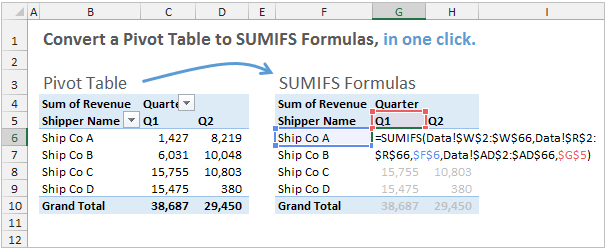## Pivot Table or SUMIFS Formulas?

Which is better – a Pivot Table or SUMIFS formulas?  That's the age-old question when creating a custom report or dashboard, and now you can have both!### Pivot Tables

The advantage of pivot tables is that you can quickly create a nicely formatted report that summarizes your data.  They are extremely fast and powerful, and save a lot of time.

The drawback is that layout of the pivot table on the worksheet can be limiting.  You may need more flexibility in the structure of the summarized data when creating custom charts, dashboards, interactive reports, etc.  Pivot tables also require the user to manually refresh them when the source data is updated.

### SUMIFS Formulas

This can lead us to use SUMIFS formulas instead of a pivot table.

The advantage of the SUMIFS formulas is that you can calculate a sum based on multiple criteria, anywhere in your spreadsheet.  They also calculate automatically when the source data is updated.

The drawback is that SUMIFS are long formulas that take a lot of time to type.  They are also more prone to errors and require more maintenance when the source data range is changed (new rows added).

## Convert Pivot Table to SUMIFS Formulas

I was recently working on a project where I was writing a lot of SUMIFS formulas to pull numbers into a dashboard.  The SUMIFS formulas are great for this because you can make the dashboard interactive using drop-downs (cell validation), and the results update automatically.

My problem was that I was spending a lot of time writing the various SUMIFS formulas.  I wanted a way to quickly create these long formulas.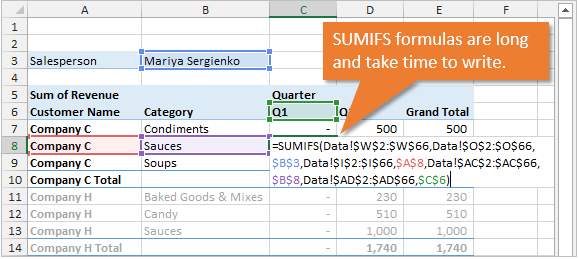So I wrote this macro that takes a Pivot Table and converts it to SUMIFS, COUNTIFS, or AVERAGEIFS formulas.  This means you can basically write the formulas using the convenience of a pivot table.  Once you have all the fields in your pivot table that are required for your SUMIFS formula, you just press a button to create the formulas.

## What Does The Macro Do Exactly?

The VBA code is below, and you can download the workbook that contains the code as well.  I also explain a few ways to use this code below.

Here is a high-level outline of what the macro does:

1. Creates a new sheet in the workbook.
2. Copies the shell of the pivot table to the new sheet (row, column, page filter areas, and formatting).
3. Loops through each cell in the values area and creates a SUMIFS, COUNTIFS, or AVERAGEIFS formula on the new sheet.  The formulas mimic the calculation of the values field in the pivot table.

You can use the new sheet as the basis for your customized report, or copy the formulas to a different sheet in the workbook.  There are a lot of ways you could use this.

## Writing the Macro

The toughest part about creating this code is handling all the potential layouts of the pivot table.  The pivot table could be in compact, outline, or tabular form, with totals and subtotals displayed in different locations.

For SUMIFS formulas, the most user-friendly layout will be Tabular with items repeating.  But I didn't want to limit the code to just that, so I tried to accommodate all the layout possibilities.

I can't say the code is perfect yet, but it does handle all the layouts I've tested so far.  I'm sure you will find errors, and I will update the code as issues arise.

## The VBA CodeConvert Pivot Table To SUMIFS Formulas.xlsm (170.6 KB)

```Dim pvt As PivotTable
Dim rSource As Range
Dim wsSource As Worksheet
Dim bTable As Boolean

Sub Convert_Pivot_to_Formulas()
'---------------------------------------------------------------------------------------
' Procedure : Convert_Pivot_to_Formulas
' Author    : Jon Acampora, Excel Campus LLC, www.excelcampus.com
' Date      : 10/13/2014
' Purpose   : Creates a copy of the selected pivot table on a new sheet and converts
'               all cells in the values area to SUMIFS, COUNTIFS, or AVERAGEIFS formulas
' Details   : The following is a list of features, requirement, and limitations of the macro.
'             Features
'               - Works with page fields with multiple items in filter of one page field.
'                   Creates the page field criteria across columns and creates array formulas.
'             Requirements
'               - Fields in the pivot containing dates must be in the same format as the source data.
'               - Source data must be in same workbook.  This can be expaned to ref source data in other workbooks.
'             Limitations
'               - Does not work with grouped date fields.  The criteria ranges do NOT exist in the source data.
'                   Create the date group fields as columns in the source data to solve this issue.
'---------------------------------------------------------------------------------------

Dim pi As PivotItem
Dim pc As PivotCell
Dim pf As PivotField
Dim wsNew As Worksheet
Dim wsPivot As Worksheet
Dim c As Range
Dim lFunction As Long
Dim sSumRange As String
Dim sCritRange As String
Dim sCriteria As String
Dim sFormula As String
Dim sFormulaPage As String
Dim sSearchField As String
Dim sDataSheet As String
Dim lDataRows As Long
Dim sPageRange As String
Dim lCol As Long
Dim bArray As Boolean
Dim sTableName As String
Dim sFormulaArgs As String
Dim sFormulaCnt As String
Dim lLblRow As Long
Dim lLblCol As Long

'Set pivot table variables
On Error Resume Next
Set pvt = ActiveCell.PivotTable
Set wsPivot = ActiveSheet
On Error GoTo 0

If pvt Is Nothing Then
MsgBox "Please select a Pivot Table first.", vbOKOnly, "Convert Pivot to Formula Error"
Exit Sub
End If

'Check if source data is in the same workbook.
If Get_Pivot_Source Then

On Error GoTo Err_Handle

'-------------------------------------------------------
'1. Create new sheet with shell of pivot table - filter, rows, columns areas
'-------------------------------------------------------
sDataSheet = wsSource.Name
lDataRows = rSource.Rows.Count
If bTable Then sTableName = pvt.SourceData

'Copy pivot table values to new sheet
wsPivot.Select
.PasteSpecial Paste:=xlPasteValues
.PasteSpecial Paste:=xlPasteFormats
.PasteSpecial Paste:=xlPasteColumnWidths
End With

If pvt.PageFields.Count > 0 Then
.PasteSpecial Paste:=xlPasteValues
.PasteSpecial Paste:=xlPasteFormats
.PasteSpecial Paste:=xlPasteColumnWidths
End With
End If

'-------------------------------------------------------
'2. Add page field filters across columns in new sheet
'-------------------------------------------------------
If pvt.PageFields.Count > 0 Then
For Each pf In pvt.PageFields
sFilter = pf.LabelRange.Offset(, 1).Resize(1, 1).Value
lCol = 0 'offset 1 col to the right
Select Case sFilter
Case "(All)"
'skip
Case "(Multiple Items)"
'Loop pivot items and add selected items to page range across columns
For Each pi In pf.PivotItems
If pi.Visible Then
wsNew.Range(sPageRange).Offset(, lCol).Resize(1, 1).Value = pi.Name
lCol = lCol + 1
End If
Next pi
Case Else 'One item selected
lCol = 1
wsPivot.Range(sPageRange).Offset(, lCol).Resize(1, 1).Value = wsNew.Range(sPageRange).Offset(, lCol).Resize(1, 1).Value
End Select

'Create string for formula
If lCol > 0 Then 'filters exist
If bTable Then
sCritRange = sTableName & "[" & pf.Name & "]"
Else
sSearchField = pf.Name
sCritRange = "'" & sDataSheet & "'!" & rSource.Resize(1).Find(What:=sSearchField, LookAt:=xlWhole).Offset(1).Resize(lDataRows - 1, 1).Address
End If

sFormulaPage = sFormulaPage & "," & sCritRange
sFormulaPage = sFormulaPage & "," & sCriteria
End If

'IFS formula will have to be an array if there are multiple critera (range) in criteria argument
If lCol > 1 Then bArray = True

Next pf
End If

'-------------------------------------------------------
'3. Loop through each cell in values area to build formula.
'-------------------------------------------------------
For Each c In pvt.DataBodyRange.Cells

Set pc = c.PivotCell
sFormula = ""
sFormulaArgs = ""

'-------------------------------------------------------
'4. Create SUM RANGE reference for formula
'-------------------------------------------------------
'Check if function is sum, count, or average
If pc.PivotField.Function = xlSum Or pc.PivotField.Function = xlCount Or pc.PivotField.Function = xlAverage Then
'Count the criteria, if 0 then it's a total and no IFS needed
sCriteria = ""

'Add column items to filter array
If pc.PivotCellType = xlPivotCellValue Then

sDataField = pc.PivotField.SourceName
lFunction = pc.PivotField.Function

lFunction = pc.PivotField.Function

If bTable Then
sSumRange = sTableName & "[" & pc.PivotField.SourceName & "]"
Else
sSearchField = pc.PivotField.SourceName
sSumRange = "'" & sDataSheet & "'!" & rSource.Resize(1).Find(What:=sSearchField, LookAt:=xlWhole).Offset(1).Resize(lDataRows - 1, 1).Address
End If

'-------------------------------------------------------
'5. Loop through ROW items of pivotcell and add row refs to formula
'-------------------------------------------------------
If pc.RowItems.Count Then
For Each pi In pc.RowItems
If bTable Then
sCritRange = sTableName & "[" & pi.Parent.Name & "]"
Else
sSearchField = pi.Parent.Name
sCritRange = "'" & sDataSheet & "'!" & rSource.Resize(1).Find(What:=sSearchField, LookAt:=xlWhole).Offset(1).Resize(lDataRows - 1, 1).Address
End If

'Start from the current row and loop up through the label range until the
'pivotitem name is found. Required due to variety of pivot table layouts.
lLblCol = pi.LabelRange.Column
For lLblRow = c.Row To pi.Parent.LabelRange.Row + 1 Step -1
If Cells(lLblRow, lLblCol).Value = pi.Name Then
Exit For
End If
Next lLblRow

If sCriteria <> "" Then
sFormulaArgs = sFormulaArgs & "," & sCritRange
sFormulaArgs = sFormulaArgs & "," & sCriteria
End If

sCriteria = ""

Next pi
End If

'-------------------------------------------------------
'6. Loop through COLUMN items of pivotcell and add row refs to formula
'-------------------------------------------------------
If pc.ColumnItems.Count Then
For Each pi In pc.ColumnItems
If bTable Then
sCritRange = sTableName & "[" & pi.Parent.Name & "]"
Else
sSearchField = pi.Parent.Name
sCritRange = "'" & sDataSheet & "'!" & rSource.Resize(1).Find(What:=sSearchField, LookAt:=xlWhole).Offset(1).Resize(lDataRows - 1, 1).Address
End If

'Start from the current column and loop back left through the label range until the
'pivotitem name is found. Required due to variety of pivot table layouts.
lLblRow = pi.LabelRange.Row
For lLblCol = c.Column To pi.LabelRange.Column Step -1
If Cells(lLblRow, lLblCol).Value = pi.Name Then
Exit For
End If
Next lLblCol

If sCriteria <> "" Then
sFormulaArgs = sFormulaArgs & "," & sCritRange
sFormulaArgs = sFormulaArgs & "," & sCriteria
End If

sCriteria = ""
Next pi
End If

'-------------------------------------------------------
'7. Build Formula based on function type of pivotcell
'-------------------------------------------------------
Select Case pc.PivotField.Function
Case xlSum
If sFormulaArgs = "" And sFormulaPage = "" Then 'Don't need IFS when there are no criteria (total rows/columns)
sFormula = "=SUM(" & sSumRange & ")"
Else
If bArray Then
sFormula = "=SUM(SUMIFS(" & sSumRange & sFormulaPage & sFormulaArgs & "))"
Else
sFormula = "=SUMIFS(" & sSumRange & sFormulaPage & sFormulaArgs & ")"
End If
End If

Case xlCount
If sFormulaArgs = "" And sFormulaPage = "" Then 'Don't need IFS when there are no criteria (total rows/columns)
sFormula = "=COUNT(" & sSumRange & ")"
Else
sFormulaCnt = sFormulaPage & sFormulaArgs 'Don't need sum range for countifs
sFormulaCnt = Right(sFormulaCnt, Len(sFormulaCnt) - 1) 'trim preceding comma

If bArray Then
sFormula = "=SUM(COUNTIFS(" & sFormulaCnt & "))"
Else
sFormula = "=COUNTIFS(" & sFormulaCnt & ")"
End If
End If

Case xlAverage
If sFormulaArgs = "" And sFormulaPage = "" Then 'Don't need IFS when there are no criteria (total rows/columns)
sFormula = "=AVERAGE(" & sSumRange & ")"
Else
sFormula = "=AVERAGEIFS(" & sSumRange & sFormulaPage & sFormulaArgs & ")" 'AVERAGEIFS not working with array formula, returns errors
End If

End Select

'-------------------------------------------------------
'8. Add formula to new sheet
'-------------------------------------------------------
If bArray Then
If Len(sFormula) < 255 Then
Else
'.FormulaArray hits an error if formula string is > 255 characters
End If
Else
End If

End If
End If
Next c

End If

wsNew.Select

Exit Sub

Err_Handle:
MsgBox Err.Description & vbNewLine & "Current Cell: " & c.Address, _
vbCritical, "Convert Pivot to Formulas Error"

End Sub

Function Get_Pivot_Source() As Boolean
Dim bReturn As Boolean
'Determine if source is a cell reference, named range, or Excel Table
'Set source range variables

'Consider using the PivotCache.SourceType property to check this.
'http://msdn.microsoft.com/en-us/library/office/ff194557.aspx

On Error GoTo Err_Handler

'Set variables for selected pivot table
bReturn = False
Set rSource = Nothing
Set wsSource = Nothing
bTable = False

If pvt.PivotCache.SourceType = xlDatabase Then
If InStr(pvt.SourceData, "[") = 0 Then 'check if source data contains workbook name - bypass external source range - Temp TO DO
If InStr(pvt.SourceData, ":") > 0 Then 'if data source range is a cell ref range
Set rSource = Application.Evaluate(Application.ConvertFormula(pvt.SourceData, xlR1C1, xlA1))
Else 'if Table or Named Range are used as data source range
Set rSource = Range(pvt.SourceData)
On Error GoTo SkipTable 'check if the data source name is a table
Set rSource = Range(pvt.SourceData & "[#All]")
bTable = True
SkipTable:
On Error GoTo 0
End If
Set wsSource = rSource.Parent
bReturn = True
End If
End If

Get_Pivot_Source = bReturn

Exit Function

Err_Handler:

MsgBox "Error in Get_Source_Range procedure."
Get_Pivot_Source = False

End Function```

## How To Implement This Code

Don't worry if all this code is making your head spin. 🙂  I have a new add-in named PivotPal coming out soon, and it will contain this feature.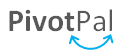This means you will be able to run this macro any time with the click of a button.  Click here to see a preview of PivotPal.

You could also add the code to your Personal Macro workbook, and then assign a ribbon button to it.  Here's an article on how to create a personal macro workbook.

In the screenshot below I added the macro to my personal macro workbook, then assigned a button to it in the Formulas tab of the ribbon.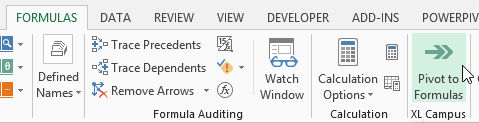You could also copy the code module into any workbook, and modify or customize it as needed.  There are a lot of possibilities with this code.

## Works With Tables and Structured References

The code also works with Excel Tables and Structured References.  If your source data range is a Table, then the code will create much nicer looking formulas.  These formulas are more dynamic when rows are added to the source data.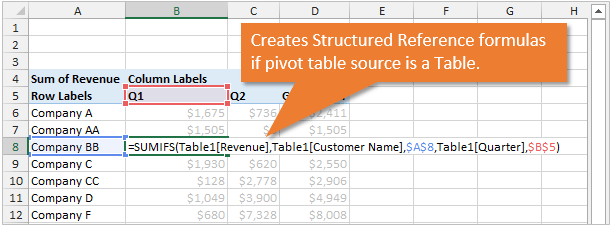This means that the formulas will automatically include any new rows that are added to the Table (source data).  Using tables will significantly cut down on the amount of maintenance your formulas will require.

## Works With Multiple Criteria Page Filter

The code will work if your pivot table has a page filter with multiple criteria selected.  The code will detect when multiple criteria are selected in the filter, and create array formulas for the SUMIFS.  It will also list the criteria out in columns to the right of the page fields.As you can see in the image above, the code works with some pretty complex layouts.  I can't guarantee that it will work in every scenario, but it should definitely cover more than the basics.

Mynda Treacy recently wrote a great article on Interactive Excel Formulas, and it explains how you can use SUMIFS formulas for your reports and dashboards.  Don't forget to checkout my free giveaways when you signup for one of Mynda's courses.

## VBA Pivot Table References

The following are a few great articles and resources for learning how to write VBA for pivot tables.  These really helped me with writing this code.Convert Pivot Table To SUMIFS Formulas.xlsm (170.6 KB)

•John DiMartino says:

I attempted to execute your code for theConvert Pivot table to SumIfs macro against a pivot table. It didn’t run without errors. I tried to debug it, but wasn’t successful. So, do you have a fixed version of the source code?

•yan says:

thank you

•Colin says:

Hi Jon,

You mention this feature would be included in Pivot Pal. Do you mean Pivot to formulas? I have Pivot Pal and I haven’t noticed this feature
Thanks

•Ishwar Singh says:

Dear Sir,

•Sarbasree dhar says:

Hi,
I need the code for MAXIF and MINIF

•Jon Acampora says:

Hi Sarbasree,

The code can be modified to use the MAXIFS and MINIFS formulas. These functions were introduced in Excel 2016 (Office 365), and will only work in that version or later. I hope that helps get you started.

•Sushil Parajuli says:

This is so awesome . Thank you very much.

•Brad says:

Hi

I did get the code working but it is not adding up criteria from the pivot that has multiple selections. Part of the SUMIFS formula that is not working
,Data!\$O\$2:\$O\$832705,\$B\$7:\$AF\$7,

If I select one, then run it, it adds up like the others.

Manually typing out a array does work, like this
Data!\$O\$2:\$O\$832705,{“HSCP”,”HSBD”}

Any suggestions? Seems more like a SUMIFS issue than your code

•Dan says:

This does not seem to work in Excel 2010 is there another way?

•mohit singh says:

hi jon
i m reaserch scholer from India.i m having 30 year data for each day.i ant to sort it to weekly basis and find summation of every week. is there any shortcut for it or i hav to do it manually. plz let me know if possible.
thank you

•kishan says:

i have an macro bill format i want to make a submit button in sheet and i want to do when submit button press all over bill data will be record in other excel sheet so kindly help me improve it

•Declan Harte says:

Hi,

I am getting an error at wsNew.Select in the add formula to new sheet section. How can I fix this?

Thanks,

Declan

‘8. Add formula to new sheet
‘——————————————————-
If bArray Then
If Len(sFormula) 255 characters
End If
Else
End If

End If
End If
Next c

End If

wsNew.Select

Exit Sub

•Jon Acampora says:

Hi Declan,
Sorry to hear that. Is it possible for you to send me your file? jon@excelcampus.com

Thanks

•Jon Acampora says:

Hi PT,

Are you using a different language version of Excel? That might be the issue.

Thanks!

•PT says:

Hi Jon,

Thanks for posting – it’s great.

I am getting the error message “Error in Get_Source_Range procedure” when I copy/paste the VBA code into my workbook.

My Excel 2007 workbook has the following:

Data tab (Table linked to Access database)
Pivot table (source is Data Tab table)

So my workbook has the source_range so I don’t know why it’s coming up with the error. What am I doing wrong? Could you please help?

Many thanks!

•Jeff Weir says:

Hey, neato! A link to this goes in my book.

•Jon Acampora says:

Thanks Jeff! I’ll need a macro to convert your book into my brain for easy reference… 🙂

•Frank says:

Jon,

this macro is what I would call sophisticated. It took me a whole day to analyse and adapt it.

There is one more limitation, I hope/guess you are aware of.

As usual VBA discriminates non-English people.

They have to replace (all), (multiple) or (blank) with the appropriate local terms.

Furthermore, the code has to be adjusted in case of dates. Cells(R,C) is localized, but pi.Name is not.

What is even worse, pvt.SourceData is localized (eg for Germany Z1S1), but Application.ConvertFormula(…, xlR1C1, xlA1) requires US-Format (R1C1).

Though I could adjust the code for my purposes (German language), I guess there is no “international” solution.

BTW, currently another minor limitation is that PageFieldOrder has to be xlDownThenOver.

Regards

Frank

•Jon Acampora says:

Hi Frank,

I’m sorry you had to spend so much time converting it. I honestly don’t have any experience programming VBA for other languages, but it sounds like it can be frustrating. If you want to send me your German version I would be happy to post it here so others can use it. jon@excelcampus.com

Please let me know if you have any other questions.
Thank you,
Jon

•Sean says:

John,

Thanks for a great post! I tried running the script against a power pivot table, and it errored out. I wasn’t surprised as the data source is not stored within an Excel sheet.

Thanks again!

Sean

•Jon Acampora says:

Hi Sean,
This macro won’t work with PowerPivot. As you said, the data source is not stored in a sheet.

However, the CUBE functions will do the same basic thing in the PowerPivot models. The CUBE functions are quite amazing, and one of my favorite features of PowerPivot. I’ve written a few blog posts on CUBEs that I will be publishing in the future.

You can actually convert a PowerPivot pivot table to cube functions with a feature built into Excel. Select any cell in a pivot table created with PowerPivot, then press the Convert to Formulas button in the OLAP Tools menu. This will convert your pivot table to CUBE formulas, so you might want to make a copy of the pivot table first.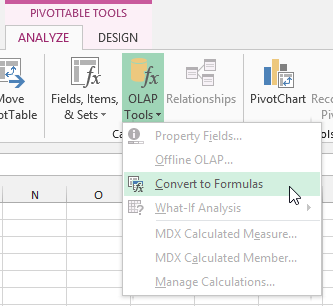Once converted, you will be able to see how the CUBE functions work. The pivot cells use the CUBEVALUE function and the row, column and filter areas use the CUBEMEMBER functions. It’s an extremely powerful and useful feature that allows you to retrieve your PowerPivot model data outside of pivot table.

The CUBEs are kind of a cross between the GETPIVOTDATA function and SUMIFS, but way more advanced and easier to use in my opinion. You can pull in any of your measures (DAX formulas), and you don’t need even need a Pivot Table.

Here are a few great resources for learning the CUBE functions.

Please let me know if you have any questions.
Thanks,
Jon

•hamid azad says:

hi’ i have no idea because can’t downlaod
i am in Iran so let me know how can i get this ebooks
very thanks

•Jon Acampora says:

Hi Hamid,
Thanks for letting me know. I will email you the eBook you receive when subscribing to my free email newsletter.
Jon

•mano says:

Dear jon
Hi,
thank you very much
kind regard – mano

•Jon Acampora says:

Thanks Mano!

•Joe Stephens says:

Hi John –

Excellent post! I’ll let you know if I encounter any issues.

A question regarding PivotPal – can it also be used with Power Pivot?

•Jon Acampora says:

Hi Joe,
Thank you and great question! PivotPal will work with Excel 2013. As I mentioned in another comment, the VBA object model does not really integrate with PowerPivot in 2010 since it was really just an add-in at that point.
Jon

•Jomili says:

I always like to see how things fail, so of course I put it through a hard test to begin with. Ran it on a workbook with Pivots based on an external data set to which I have no access. The code errored out, going to the “wsNew.Select” code in Step 8. Gave me a “Run-Time Error 91”.

•Jon Acampora says:

Hi Jomili,
The macro will only work when the pivot table is in the same workbook as the source data. This could be enhanced to work with pivots where the source data is in another workbook, but you would need access to the external file as it would have to be open when the code runs.

This is required because the SUMIFS formula is pointing to the source data, and the macro needs to access the source data to find the criteria range columns when creating the formulas.
Please let me know if you have any questions.
Thanks,
Jon

•Jomili says:

Suggested tweak: add msgbox to note if Data Source is external rather than have the macro error out.

Generic filters
Exact matches only
Filter by Custom Post TypeLearn 10 great Excel techniques that will wow your boss and make your co-workers say, "how did you do that??"